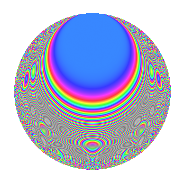# Properties

 Label 96.2.oLevel 96 Weight 2 Character orbit o Rep. character $$\chi_{96}(11,\cdot)$$ Character field $$\Q(\zeta_{8})$$ Dimension 56 Newform subspaces 1 Sturm bound 32 Trace bound 0

# Related objects

## Defining parameters

 Level: $$N$$ = $$96 = 2^{5} \cdot 3$$ Weight: $$k$$ = $$2$$ Character orbit: $$[\chi]$$ = 96.o (of order $$8$$ and degree $$4$$) Character conductor: $$\operatorname{cond}(\chi)$$ = $$96$$ Character field: $$\Q(\zeta_{8})$$ Newform subspaces: $$1$$ Sturm bound: $$32$$ Trace bound: $$0$$

## Dimensions

The following table gives the dimensions of various subspaces of $$M_{2}(96, [\chi])$$.

Total New Old
Modular forms 72 72 0
Cusp forms 56 56 0
Eisenstein series 16 16 0

## Trace form

 $$56q - 4q^{3} - 8q^{4} - 4q^{6} - 8q^{7} - 4q^{9} + O(q^{10})$$ $$56q - 4q^{3} - 8q^{4} - 4q^{6} - 8q^{7} - 4q^{9} - 16q^{10} - 4q^{12} - 8q^{13} - 8q^{15} - 40q^{16} - 4q^{18} - 8q^{19} - 4q^{21} - 24q^{22} + 16q^{24} - 8q^{25} - 28q^{27} - 8q^{28} + 44q^{30} - 8q^{33} - 24q^{34} + 48q^{36} - 8q^{37} - 28q^{39} + 24q^{40} + 56q^{42} - 8q^{43} - 4q^{45} + 24q^{46} + 48q^{48} - 16q^{51} + 48q^{52} + 40q^{54} + 24q^{55} - 4q^{57} + 96q^{58} + 8q^{60} - 40q^{61} + 40q^{64} - 28q^{66} + 56q^{67} - 4q^{69} + 40q^{70} - 64q^{72} - 8q^{73} + 16q^{75} + 48q^{76} - 92q^{78} + 16q^{79} - 48q^{82} - 136q^{84} - 48q^{85} + 52q^{87} - 48q^{88} - 136q^{90} + 40q^{91} + 8q^{93} - 64q^{94} - 104q^{96} - 16q^{97} + 60q^{99} + O(q^{100})$$

## Decomposition of $$S_{2}^{\mathrm{new}}(96, [\chi])$$ into newform subspaces

Label Dim. $$A$$ Field CM Traces $q$-expansion
$$a_2$$ $$a_3$$ $$a_5$$ $$a_7$$
96.2.o.a $$56$$ $$0.767$$ None $$0$$ $$-4$$ $$0$$ $$-8$$

## Hecke Characteristic Polynomials

There are no characteristic polynomials of Hecke operators in the database